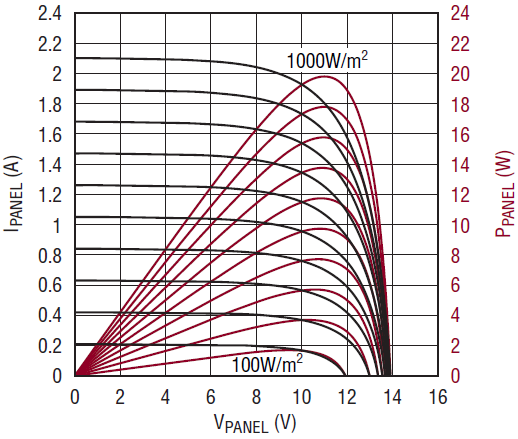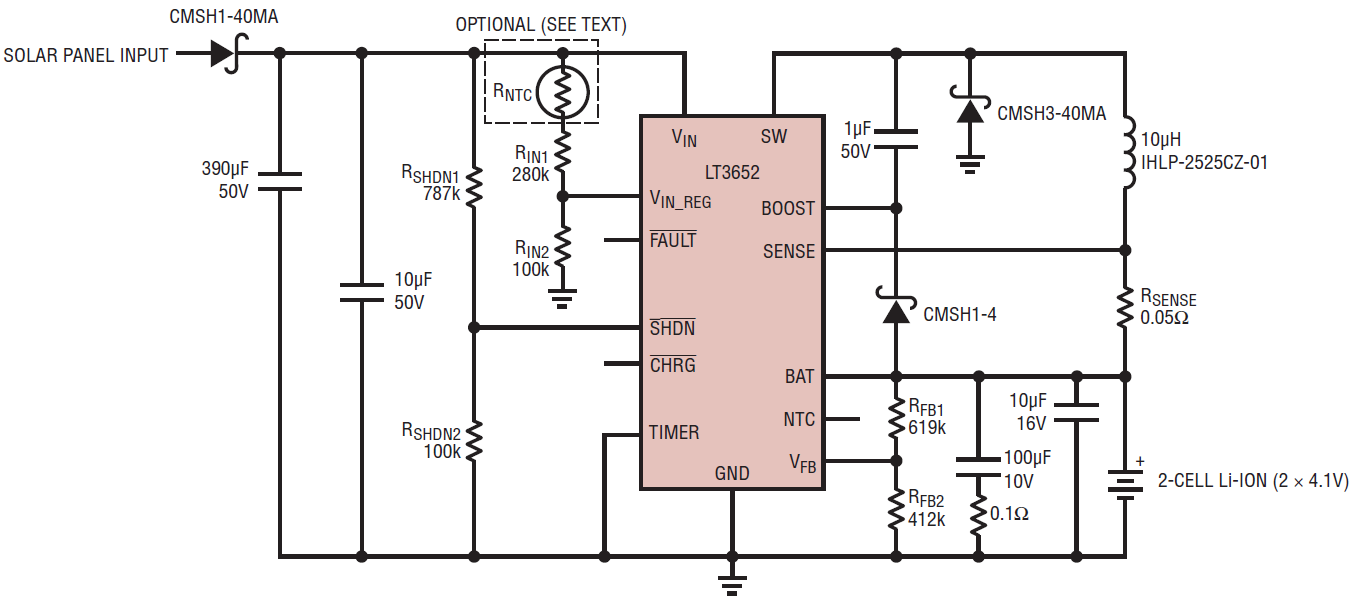Designing a Solar Cell Battery Charger

Introduction

The market for portable solar powered electronic devices continues to grow as consumers look for ways to reduce energy consumption and spend more time outdoors. Because solar power is a variable and unreliable, nearly all solar-powered devices feature rechargeable batteries. The goal is to extract as much solar power as possible to charge the batteries quickly and maintain the charge.

Solar cells are inherently inefficient devices, but they do have a point of maximum power output, so operating at that point seems an obvious design goal. The problem is that the IV characteristic of maximum output power changes with illumination. A monocrystalline solar cell’s output current is proportional to light intensity, while its voltage at maximum power output is relatively constant (see Figure 1). Maximum power output for a given light intensity occurs at the knee of each curve, where the cell transitions from a constant voltage device to a constant current device. A charger design that efficiently extracts power from a solar panel must be able to steer the panel’s output voltage to the point of maximum power when illumination levels cannot support the charger’s full power requirements.Figure 1. A solar cell produces current in proportion to the amount of sunlight falling on it, while the cell’s open-circuit voltage remains relatively constant. Maximum power output occurs at the knee of each curve, where the cell transitions from a constant voltage device to a constant current device, as shown by the power curves.

The LT3652 is a multi-chemistry 2A battery charger designed for solar power applications. The LT3652 employs an input voltage regulation loop that reduces the charge current if the input voltage falls below a programmed level set by a simple voltage divider network. When powered by a solar panel, the input voltage regulation loop is used to maintain the panel at near peak power output.

LT3652 Input Voltage Regulation Loop

The input voltage regulation loop of the LT3652 acts over a specific input voltage range. When VIN, as measured via a resistor divider at the VIN_REG pin, falls below a certain set point, the charge current is reduced. The charging current is adjusted via a control voltage across a current sensing resistor in series with the inductor of the buck regulator charging circuit. Decreased illumination (and/or increased charge current demands) can both cause the input voltage (panel voltage) to fall, pushing the panel away from its point of maximum power output. With the LT3652, when the input voltage falls below a certain set point, as defined by the resistor divider connected between the VIN and VIN_REG pins, the current control voltage is reduced, thus reducing the charging current. This action causes the voltage from the solar panel to increase along its characteristic VI curve until a new peak power operating point is found.

If the solar panel is illuminated enough to provide more power than is required by the LT3652 charging circuit, the voltage from the solar panel increases beyond the control range of the voltage regulation loop, the charging current is set to its maximum value and a new operation point is found based entirely on the maximum charging current for the battery’s point in the charge cycle.

If the electronic device is operating directly from solar power and the input voltage is above the minimum level of the input voltage regulation loop’s control range, the excess power available is used to charge the battery at a lower charging rate. The power from the solar panel is adjusted to its maximum operating power point for the intensity level.Figure 2. Charger current control voltage (VSENSE – VBAT) vs proportional input voltage, as measured via voltage divider at VIN_REG pin. VIN (solar panel voltage) only affects charging current when VIN_REG is between 2.67V and 2.74V. In this range, the charger will reduce the charging current if necessary to run the panel at peak power output.

Figure 2 shows a typical VIN_REG control characteristic curve. As the voltage on the VIN_REG pin increases beyond 2.67V, the voltage VSENSE – VBAT, across the current sensing resistor, increases until it reaches a maximum of 100mV, when VIN_REG is above 2.74V. As VIN_REG increases further, VSENSE – VBAT remains at 100mV. The expression for the input voltage control range is:

If we linearize the portion of the curve in Figure 2 for VIN_REG between 2.67V and 2.74V, the following expression describes the current sensing voltage VSENSE – VBAT:

The charging current for the battery would then be:

Since the charging circuit of the LT3652 is a current controlled buck regulator, the input current relates to the charging current by the following expression:

where η is the efficiency of the charger

The input power can now be determined by combining Equations 4 and 5 with the input voltage, resulting in the following:

Once RSENSE is selected for the maximum charging current and RIN1 and RIN2 are determined to select the input voltage current control range, Equation 6 can be plotted against the solar panels power curves to determine the charger’s operating point for various battery voltages. An example follows.

Design Example

Figure 3 shows a 2A, solar powered, 2-cell Li-Ion battery charger using the LT3652.Figure 3. 2A Solar-powered battery charger.

First step is to determine the minimum requirements for the solar panel. Important parameters include the open circuit voltage, VOC, peak power voltage, VP(MAX), and peak power current, IP(MAX). The short circuit current, ISC, of the solar panel falls out of the calculations based on the other three parameters.

The open circuit voltage must be 3.3V plus the forward voltage drop of D1 above the float voltage of the 2-cell Li-ion battery plus an additional 15% for low intensity start-up and operation.

The peak power voltage must be 0.75V plus the forward drop of D1 above the float voltage plus an additional 15% for low intensity operation.

The peak input power current is the product of the float voltage and the maximum charging current divided by the peak power input voltage and the efficiency of the charging circuit.

Solving for these three equations, we can define the minimum requirements of the solar panel:

The solar panel characteristics can be seen in Figure 4.Figure 4. Action of the solar battery charger circuit in Figure 3. Power-intensity curves for various illumination levels are shown for 100W/m2 to 1000W/m2 in 100W/m2 steps. The VIN control range (VREG) is also shown. The VIN control loop extracts maximum possible power from the solar panel by steering VIN to the top of the panel’s power-intensity curve when VIN is in the VREG range.

The current sensing resistor, RSENSE, is determined from the maximum VSENSE – VBAT of 100mV divided by the maximum charging current of 2A

The output feedback voltage divider network of RFB1 and RFB2 are determined next. The voltage divider network must have a Thevenin’s equivalent resistance of 250k to compensate for input bias current error. The VFB pin reference voltage is 3.3V.

The next step is to set the peak power tracking voltage using the voltage divider network of RIN1 and RIN2 connected between the VIN and the VIN_REG pins.

Verify the minimum and maximum peak power input tracking voltages.

The final step in selecting resistor values is to determine the VSHDN voltage divider network consisting of RSHDN1 and RSHDN2. The VSHDN rising threshold is 1.2V ± 50mV with a hysteresis of 120mV. The voltage divider network wants to be set such that, when the voltage on the VIN pin is at VREG(MIN), VSHDN is at its maximum possible value.

The VSHDN limits are now determined as:

The LT3652 automatically enters a battery precondition mode if the sensed battery voltage is very low. In this mode, the charge current is reduced to 15% of the programmed maximum, as set by the current sensing resistor, RSENSE. Once the battery voltage reaches 70% of the fully charged float voltage (VFB = 2.3V), the LT3652 automatically increases maximum charge current to the full programmed value. The battery voltage threshold level between precondition mode and maximum charge current is determined as follows:

Using and efficiency of 0.85, plot PIN over the range of VIN that is current controlled. This is the regulated VIN, or VREG, power line. The intersection of the VREG power line with the solar panel power curve is the operating point. As the battery charges, the slope of the VREG power line increases, indicating the increase in input power required to support the increasing output power. The intersection of the VREG power line continues to follow up the solar panel’s power curves until the charger exits constant current mode.

The resulting plots are shown in Figure 4.

The Circuit in Action

Figure 4 shows the power output of the solar panel plotted at light intensity levels from 100W/m2 to 1000W/m2 in 100W/m2 steps. At maximum light intensity (top curve in Figure 4) and the battery voltage just above the preconditioning level (VBAT(MIN) at 2A), the solar panel is producing more power than the charger needs. The solar panel voltage rises above the VREG control voltage and travels across the constant power line until it intersects the light-power-intensity curve for that intensity level (point A in Figure 4). As the battery charges, the input power increases and the solar panel operating point moves up the lightpower-intensity curve until the battery approaches full charge (point B). The LT3652 transitions from constant current mode to constant voltage mode and the charging current is reduced. The solar panel operating point moves back down the light-power-intensity curve to the open circuit voltage (point C) when the battery reaches its final float voltage.

During the charging of the battery, if the light intensity diminishes, the operation point moves across a constant power line for the battery voltage until it reaches the new power-intensity curve. If the light intensity level continues to diminish, the operating point travels along this constant power line until it reaches the VREG power line. At this point the charging current is reduced until the operating point is at the intersection of the light-power-intensity curve and the VREG power line (point D for constant current charging at VBAT(FLOAT) with 800W/m2 illumination). As the battery continues to charge at this light intensity level, the operating point moves along the new light-power-intensity curve until the battery approaches full charge.

As darkness approaches, the operating point moves down the VREG power line until charging current ceases (point E) and the solar panel output voltage drops below the SHDN falling threshold at which point the LT3652 turns off.

The remaining elements of the design, selection of output inductor, catch rectifier and timer capacitor, are outlined in the design procedure in the LT3652 datasheet along with PCB layout considerations.

The maximum power voltage, for a monocrystalline solar cell, has a temperature coefficient of –0.37%/K while the maximum power level is –0.47%/K. This may be compensated for by letting RIN1 be a combination of a series resistor and a series NTC thermistor. The ratio of the two elements that comprise RIN1 and the value of RIN2 need to be adjusted to achieve the correct negative temperature of VIN while still maintaining the control range of VIN.

Conclusion

The input voltage regulation loop of the LT3652 has the ability to seek out the maximum power operating point of a solar panel’s power characteristic, thus utilizing the full capacity of the solar panel. The float voltage regulation loop and its adjustable charging current enable the LT3652 to be used with many battery chemistries, making it a versatile battery charger. The added features of a wide input voltage range, an auto-recharge cycle to maintain a fully charged battery, a battery preconditioning mode, NTC temperature sensing, selectable C/10 or timed charging termination, a FAULT and a charging status pins fills out the full feature set of the LT3652. The LT3652 is available in a 3mm × 3mm 12-lead plastic DFN, package with an exposed pad.